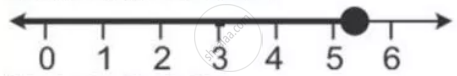Share

Solve and Graph the Solution Set Of: 2x – 9 < 7 and 3x + 9 ≤ 25, X ∈ R - ICSE Class 10 - Mathematics

ConceptLinear Inequations in One Unknown

Question

Solve and graph the solution set of:

2x – 9 < 7 and 3x + 9 ≤ 25, x ∈ R

Solution

2x - 9 < 7 and 3x + 9 <= 25

2x  > 16 and 3x  <= 16

x < 8 and x <=  5 1/3

∴ Solution set = {x <= 5 1/3, x ∈ R}

The required graph on number line is:Is there an error in this question or solution?

APPEARS IN

Solution Solve and Graph the Solution Set Of: 2x – 9 < 7 and 3x + 9 ≤ 25, X ∈ R Concept: Linear Inequations in One Unknown.
S